# 20192221 2019-2020-2 《Python程序设计》实验四报告

## 实验内容选择

Python综合应用：爬虫、数据处理、可视化、机器学习、神经网络、游戏、网络安全等。

## 2.实验过程及结果

### 1.找到弹幕对应的网页api（F12，然后找list.so?oid）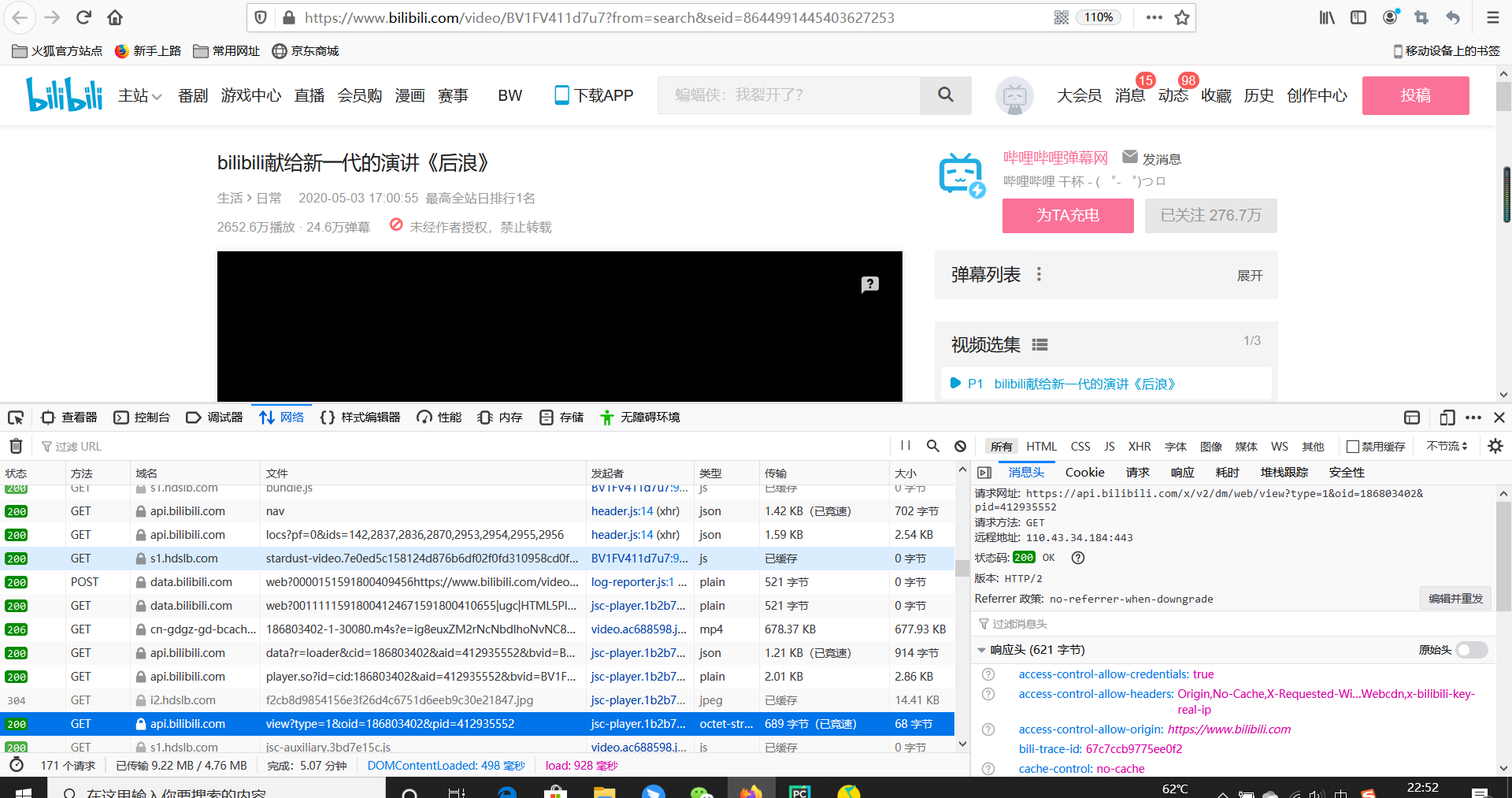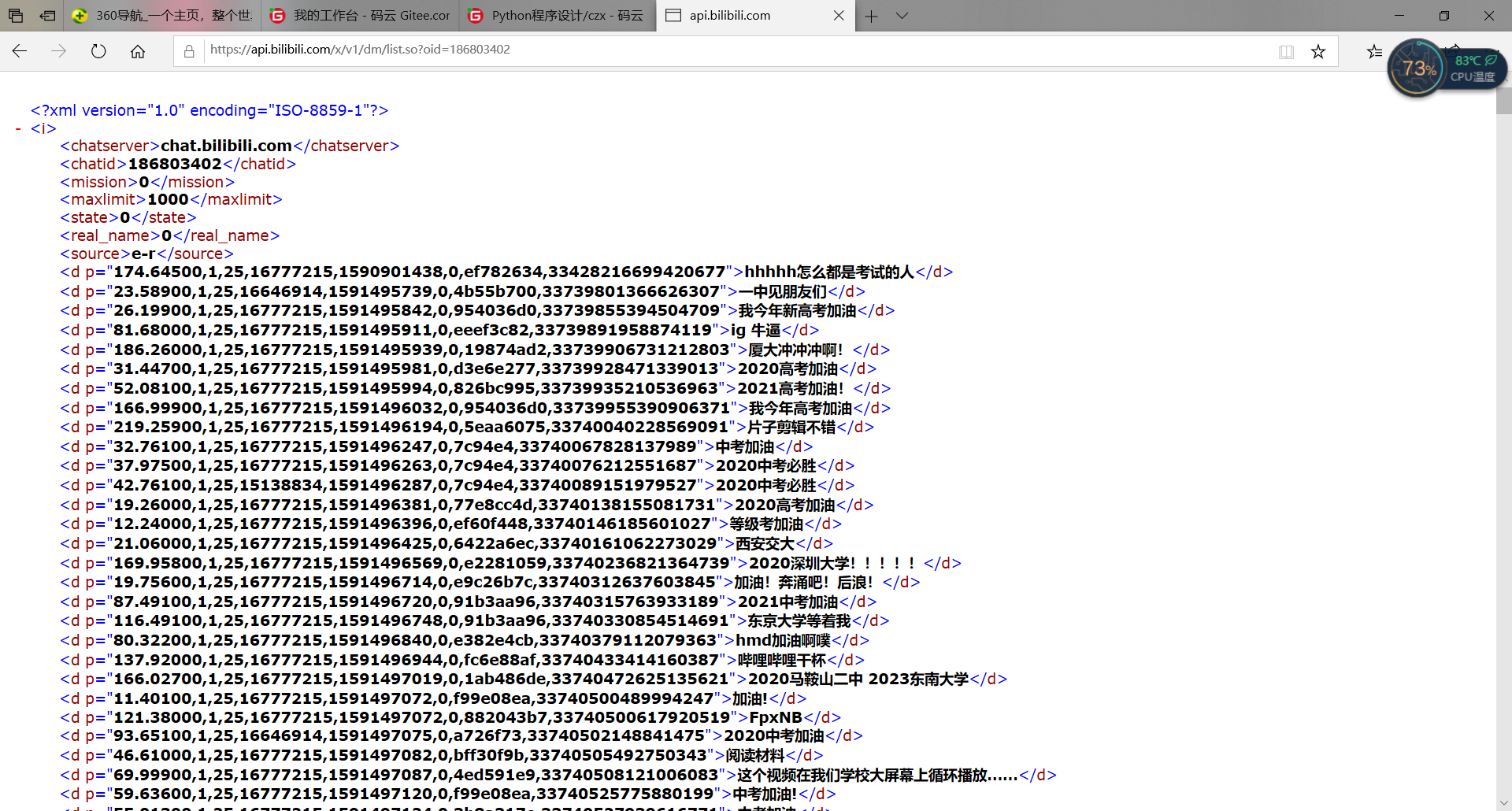### 2.获取我们需要的信息

###### requests.get → 解码 → 正则表达式提取（这里没必要使用beautifulsoup，故没用了）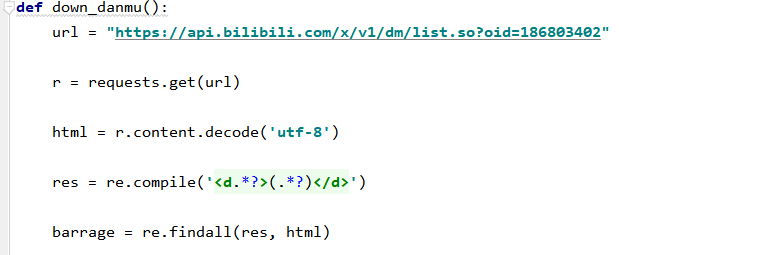### 3.存入CSV文件和XLS文件中

##### xls：直接创建一个workbook和worksheet，然后逐条写入（因为太多了，所以我只存了前500条）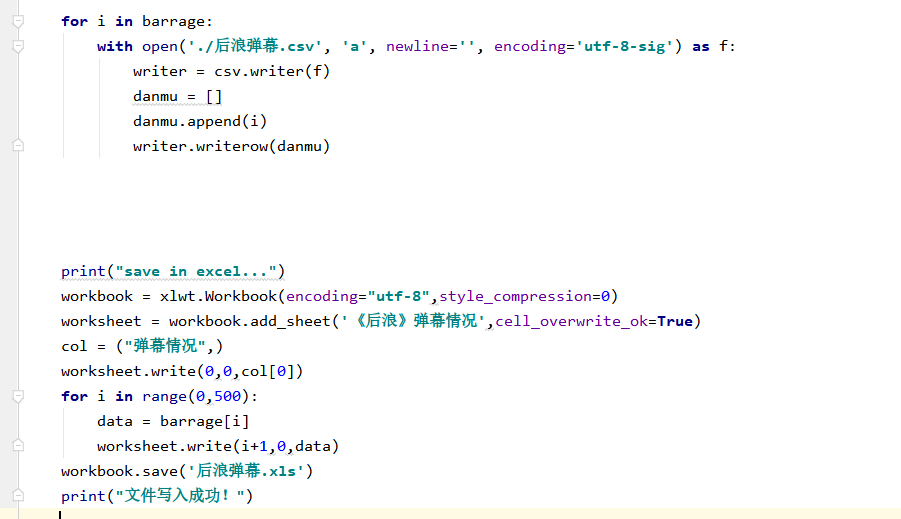### 4.制作成词云

##### → 设置生成词云的参数（颜色、字体等等） → 加入词语，保存图片文件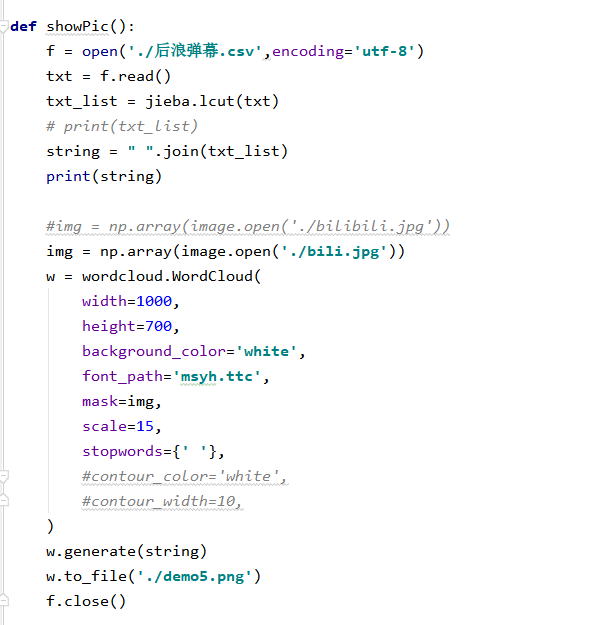### 5.源代码



import requests
import re
import csv
import jieba
import wordcloud
import PIL.Image as image
import numpy as np
import xlwt

def down_danmu():
url = "https://api.bilibili.com/x/v1/dm/list.so?oid=186803402"

r = requests.get(url)

html = r.content.decode('utf-8')

res = re.compile('<d.*?>(.*?)</d>')

barrage = re.findall(res, html)

for i in barrage:
with open('./后浪弹幕.csv', 'a', newline='', encoding='utf-8-sig') as f:
writer = csv.writer(f)
danmu = []
danmu.append(i)
writer.writerow(danmu)

print("save in excel...")
workbook = xlwt.Workbook(encoding="utf-8",style_compression=0)
worksheet = workbook.add_sheet('《后浪》弹幕情况',cell_overwrite_ok=True)
col = ("弹幕情况",)
worksheet.write(0,0,col)
for i in range(0,500):
data = barrage[i]
worksheet.write(i+1,0,data)
workbook.save('后浪弹幕.xls')
print("文件写入成功！")


def showPic():
f = open('./后浪弹幕.csv',encoding='utf-8')
txt = f.read()
txt_list = jieba.lcut(txt)
# print(txt_list)
string = " ".join(txt_list)
print(string)

#img = np.array(image.open('./bilibili.jpg'))
img = np.array(image.open('./bili.jpg'))
w = wordcloud.WordCloud(
width=1000,
height=700,
background_color='white',
font_path='msyh.ttc',
mask=img,
scale=15,
stopwords={' '},
#contour_color='white',
#contour_width=10,
)
w.generate(string)
w.to_file('./demo5.png')
f.close()


down_danmu()
showPic()

### 7.成果展示：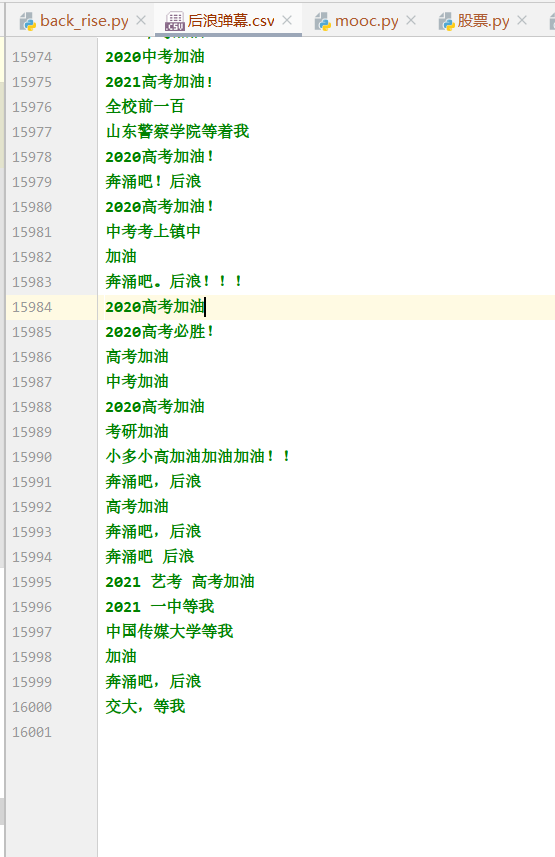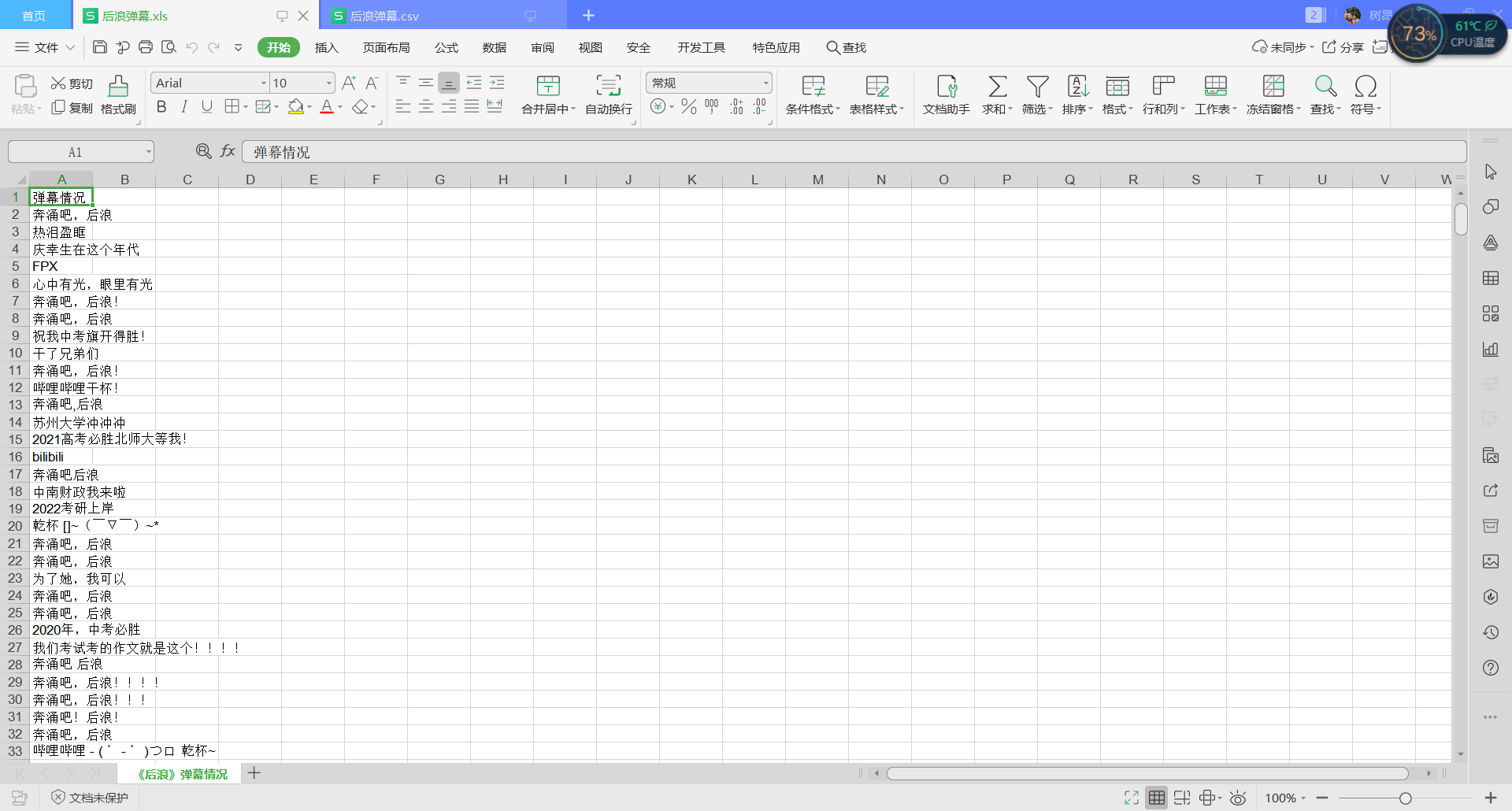## 其他（感悟、思考等）

### 建议：

①以后还是像后面那样课程中讲一遍内容吧，也算是对网上视频内容的复习。
②希望以后课程中的例子能更生动活泼一点，要是能紧跟潮流就更好了，比如说在涉及文本内容时写一些当红游戏，网络流行语什么的。
③可以课程中能讲解一些项目。